Question

# Determine the magnitude of the force on an electron traveling 8.31E+5 m/s horizontally to the east...

Determine the magnitude of the force on an electron traveling 8.31E+5 m/s horizontally to the east in a vertically upward magnetic field of strength 0.800 T.
(in N)

 A: 2.79

F = q(vxB)

As v and B are perpendicular to each other hence F = 1.6E-19*8.31E5*0.8 = 1.06368 E -13

Hence option G is correct

B: 3.49

G: 1.06

The magnetic force on the electron is,

F = Bvq

= (0.8 T)(8.31x105 m/s)(1.6x10-19 C)

= 1.06x10-13 N

G) 1.06*10^-13 N

F = q*v*B*sin(90)

= 1.6*10^-19*8.31*10^5*0.8*1

= 1.06*10^-13 N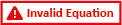G : 1.06*10^-13

#### Earn Coins

Coins can be redeemed for fabulous gifts.

Similar Homework Help Questions
• ### Determine the magnitude of the force on an electron traveling 6.05×105 m/s horizontally to the east...

Determine the magnitude of the force on an electron traveling 6.05×105 m/s horizontally to the east in a vertically upward magnetic field of strength 0.35 T . Determine the direction of the force on an electron.

• ### Determine the magnitude of the force on an electron traveling 7.20×105 m/s horizontally to the east...

Determine the magnitude of the force on an electron traveling 7.20×105 m/s horizontally to the east in a vertically upward magnetic field of strength 0.59 T .

• ### Determine the magnitude of the force on an electron traveling 5.95×105 m/s horizontally to the east...

Determine the magnitude of the force on an electron traveling 5.95×105 m/s horizontally to the east in a vertically upward magnetic field of strength 0.28 T

• ### Determine the magnitude of the force on an electron traveling 6.15×105 m/s horizontally to the east...

Determine the magnitude of the force on an electron traveling 6.15×105 m/s horizontally to the east in a vertically upward magnetic field of strength 0.80 T . Which Direction ?

• ### Determine the magnitude of the force on an electron traveling 6.35×10^5 m/s horizontally to the east in a vertically upward magnetic field?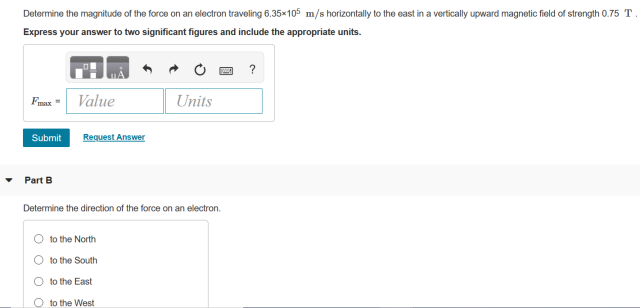A) Determine the magnitude of the force on an electron traveling 6.35×10^5 m/s horizontally to the east in a vertically upward magnetic field of strength 0.75 T.B) Determine the direction of the force on an electron.     1. to the North     2. to the South     3. to the East     4. to the West

• ### A. Determine the magnitude of the force on an electron traveling 5.40×105 m/s horizontally to the...

A. Determine the magnitude of the force on an electron traveling 5.40×105 m/s horizontally to the east in a vertically upward magnetic field of strength 0.33 T . B. Determine the direction of the force on an electron.

• ### Determine the magnitude and direction of the force on an electron traveling 8.75x105 m/s horizontally to...

Determine the magnitude and direction of the force on an electron traveling 8.75x105 m/s horizontally to the east in a vertically upward magnetic field of strength 0.75 T.

• ### Determine the magnitude of the force on an electron traveling 6.45-105 m/s horizontally to the east...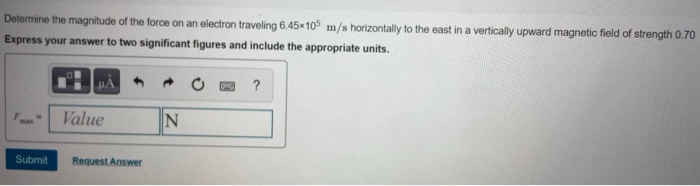Determine the magnitude of the force on an electron traveling 6.45-105 m/s horizontally to the east in a vertically upward magnetic field of strength 0.70 Express your answer to two significant figures and include the appropriate units. JA → ? F Value N Submit Request Answer Part B Determine the direction of the force on an electron. O to the North to the South O to the East O to the West Submit Request Answer

• ### Consta Part A Determine the magnitude of the force on an electron traveling 6.15x109 m/s horizontally...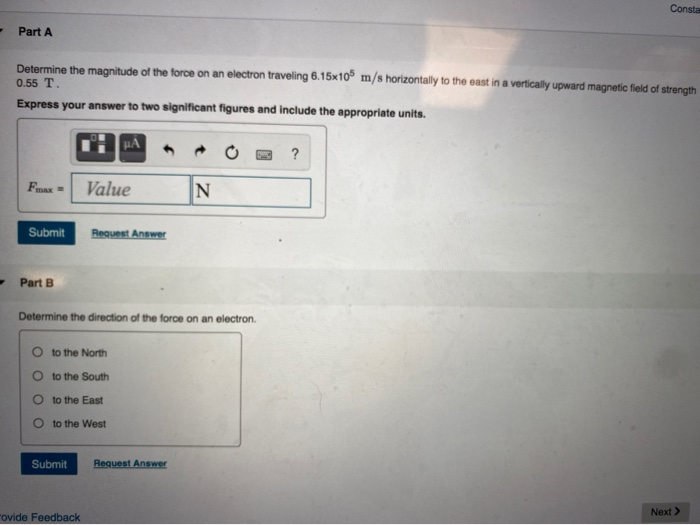Consta Part A Determine the magnitude of the force on an electron traveling 6.15x109 m/s horizontally to the east in a vertically upward magnetic field of strength 0.55 T. Express your answer to two significant figures and include the appropriate units. NA ? Fmax = Value N Submit Request Answer - Part B Determine the direction of the force on an electron to the North to the South O to the East O to the West Submit Request Answer ovide...

• ### Determine the magnitude and direction of the force on an electron traveling 8.75 x 105m/s horizontally...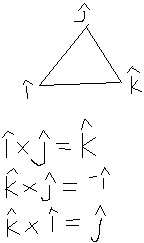Determine the magnitude and direction of the force on an electron traveling 8.75 x 105m/s horizontally to the east in a vertically upward magnetic field of strength 0.45 T. I got that v= v j <----vector and B= B k <----vector, but how do i know this??? The answer is F= (-e) v x B F= -e v B i <-----why i is positive when in the diagram above k x j = -i ??? F= -1.6 x 10-19 x...